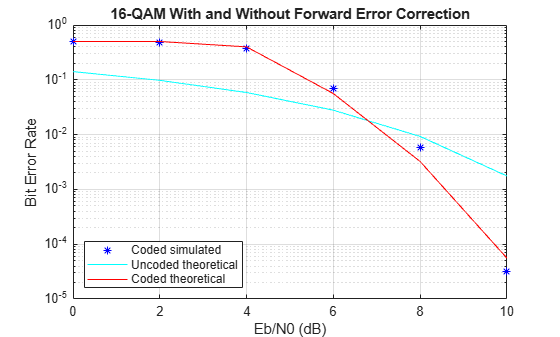# Rate 2/3 Convolutional Code in AWGN

This example generates a bit error rate versus ${\mathit{E}}_{\mathit{b}}/{\mathit{N}}_{0}$ curve for a link that uses 16-QAM modulation and a rate 2/3 convolutional code in AWGN.

Set the modulation order, and compute the number of bits per symbol.

```M = 16; k = log2(M);```

Create a trellis for a rate 2/3 convolutional code. Set the traceback and code rate parameters.

```trellis = poly2trellis([5 4],[23 35 0; 0 5 13]); traceBack = 28; codeRate = 2/3;```

Create a convolutional encoder and its equivalent Viterbi decoder to run in the continuous mode.

```convEncoder = comm.ConvolutionalEncoder(TrellisStructure=trellis); vitDecoder = comm.ViterbiDecoder( ... TrellisStructure=trellis, ... InputFormat='Hard', ... TracebackDepth=traceBack);```

Create an error rate object. Set the receiver delay to twice the traceback depth, which is the delay through the decoder.

`errorRate = comm.ErrorRate(ReceiveDelay=2*traceBack);`

Set the range of ${\mathit{E}}_{\mathit{b}}/{\mathit{N}}_{0}$ values to be simulated and compute the equivalent SNR values. Initialize the bit error rate statistics matrix.

```ebnoVec = 0:2:10; snr = convertSNR(ebnoVec,"ebno","snr", ... BitsPerSymbol=k, ... CodingRate=codeRate); errorStats = zeros(length(ebnoVec),3);```

Simulate the link by following these steps:

• Generate binary data.

• Encode the data with a rate 2/3 convolutional code.

• 16-QAM modulate the encoded data, configure bit inputs and unit average power.

• Pass the signal through an AWGN channel.

• 16-QAM demodulate the received signal configure bit outputs and unit average power.

• Decode the demodulated signal by using a Viterbi decoder.

• Collect the error statistics.

```for ii = 1:length(ebnoVec) while errorStats(ii,2) <= 100 && errorStats(ii,3) <= 1e7 dataIn = randi([0 1],10000,1); dataEnc = convEncoder(dataIn); txSig = qammod(dataEnc,M, ... InputType='bit',UnitAveragePower=true); rxSig = awgn(txSig,snr(ii),'measured'); demodSig = qamdemod(rxSig,M, ... OutputType='bit',UnitAveragePower=true); dataOut = vitDecoder(demodSig); errorStats(ii,:) = errorRate(dataIn,dataOut); end reset(errorRate) end```

Compute the theoretical BER curve for the case without forward error correction coding by using the `berawgn` function.

`berUncoded_emp = berawgn(ebnoVec','qam',M);`

Compute the theoretical BER curve for the case with forward error correction coding by using the `bercoding` function and the distance spectrum for the 2/3 rate convolutional code. The `distspec` function computes the distance spectrum of convolutional codes and outputs the distance spectrum structure.

`spect = distspec(trellis,4)`
```spect = struct with fields: dfree: 5 weight: [1 6 28 142] event: [1 2 8 25] ```
```berCoded_emp = bercoding(ebnoVec', ... 'conv','hard',codeRate,spect,'qam',M);```

Plot BER versus ${\mathit{E}}_{\mathit{b}}/{\mathit{N}}_{0}$ curves for the simulated coded data, and the theoretical uncoded and coded data. At higher ${\mathit{E}}_{\mathit{b}}/{\mathit{N}}_{0}$ values, the error correcting code provides performance benefits. The simulated coded error rate results show good correlation to the theoretical coded results.

```semilogy(ebnoVec,errorStats(:,1),'b*', ... ebnoVec,berUncoded_emp,'c-', ... ebnoVec,berCoded_emp,'r') grid legend('Coded simulated','Uncoded theoretical','Coded theoretical', ... 'Location','southwest') title('16-QAM With and Without Forward Error Correction') xlabel('Eb/N0 (dB)') ylabel('Bit Error Rate')```